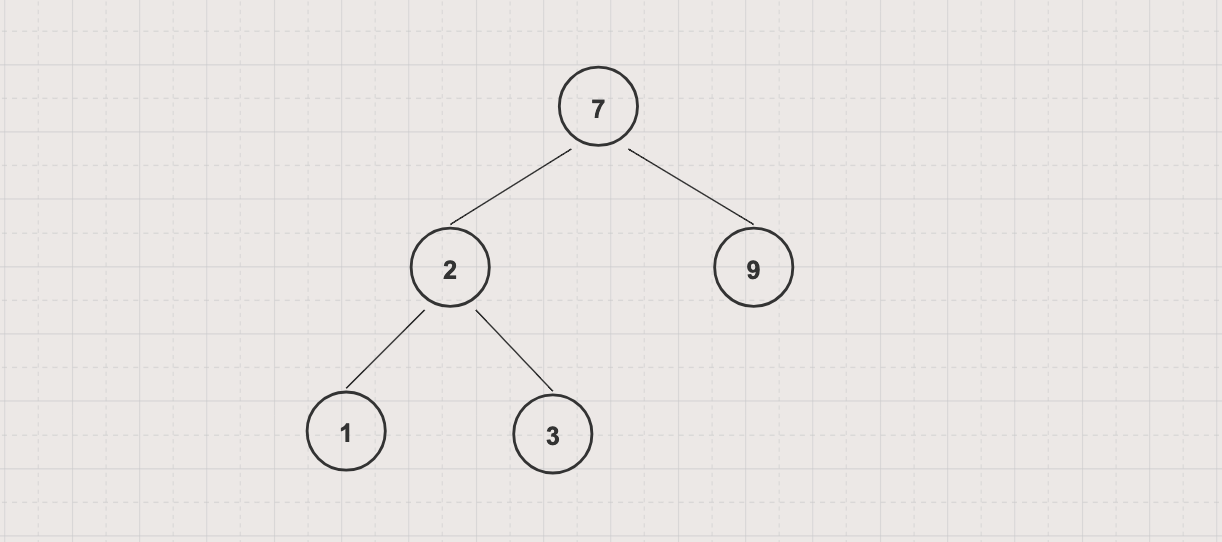Tree traversal is a process of visiting each node in a tree exactly once. Unlike linear data structures such as an array or linked list which is canonically traversed in linear order, a tree may be traversed in depth-first or breadth-first order

Breadth-first traversal visits all nodes on the same level before going to another lower levelFor the above tree, assuming that the left subtree are chosen before the right subtree, breadth-first traversal orders will be 7-2-9-1-3

## Implementation example on a binary tree

We can follow these steps to implement a bread first traversal algorithm on a binary tree

• Create a queue Q to track the traversal path

• Add the root node into Q

• Do the following while Q still contains at least one node

• Remove a node N from Q

• Print the value of N

• Add left child of N into Q if it is not null

• Add right child of N into Q if it is not null

``````import java.util.ArrayDeque;
import java.util.Queue;

queue.offer(root);

while (!queue.isEmpty()) {
System.out.println(currentNode);

if (currentNode.left != null)
queue.offer(currentNode.left);

if (currentNode.right != null)
queue.offer(currentNode.right);
}
}

public static void main(String[] args) {
tree.insert(7);
tree.insert(2);
tree.insert(3);
tree.insert(1);
tree.insert(9);

}
}
``````

Output

``````data: 7, left: 2, right: 9
data: 2, left: 1, right: 3
data: 9, left: null, right: null
data: 1, left: null, right: null
data: 3, left: null, right: null
``````

`BSTByLinkedList` is defined in Binary Search Tree Data Structure